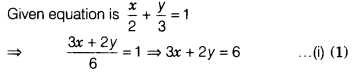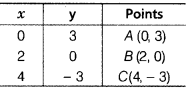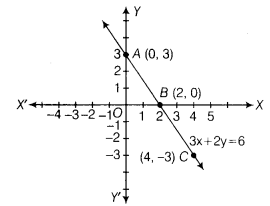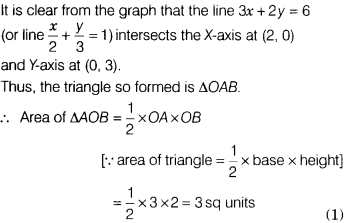# Draw the graph of the straight line given by the equation x/2+y/3=1

Draw the graph of the straight line given by the equation x/2+y/3=1. Calculate the area of triangle formed by the line drawn and the coordinate axisOn putting x = 0 in Eq. (i), we get
3 × 0 +2y = 6 => y = 3
On putting x = 2 in Eq, (i), we get
6 + 2y = 6 => y = 0
On putting x = 4 in Eq. (i), we get
12 + 2y = 6 => 2y = -6 => y = -3
Thus, we get the following tableNow, plot the points A(0, 3), 6(2, 0) and C(4, - 3) on the graph paper and join them to get a line AC which represents the graph of the equation 3x + 2y = 6.